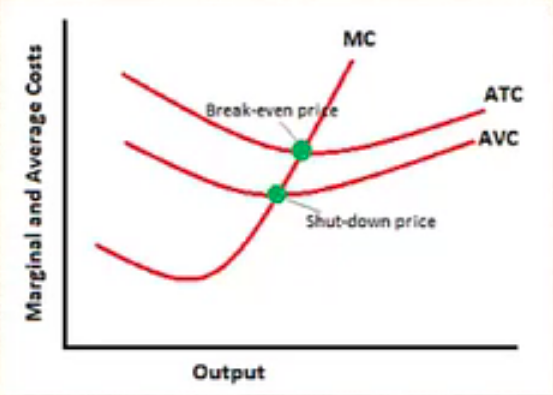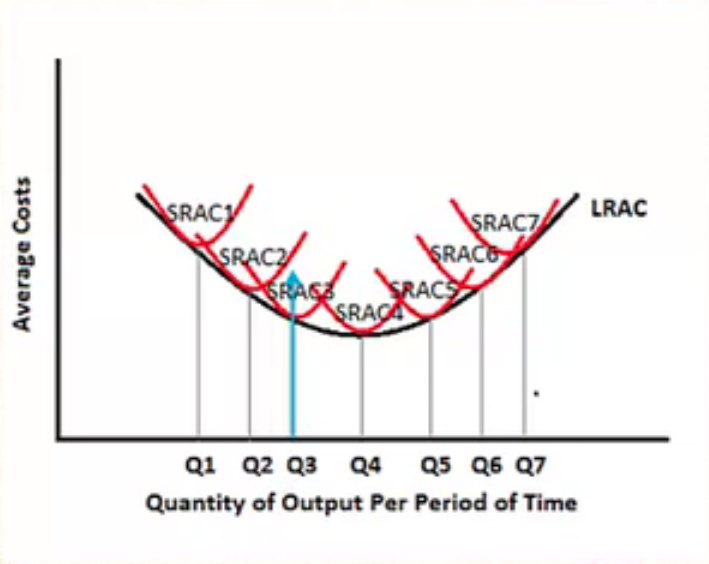+

# Long Run Supply Curve

Author: Sophia Tutorial
##### Description:

Determine the relationship between short-run and long-run average cost curves.

(more)### Developing Effective Teams

*No strings attached. This college course is 100% free and is worth 1 semester credit.

46 Sophia partners guarantee credit transfer.

299 Institutions have accepted or given pre-approval for credit transfer.

* The American Council on Education's College Credit Recommendation Service (ACE Credit®) has evaluated and recommended college credit for 33 of Sophia’s online courses. Many different colleges and universities consider ACE CREDIT recommendations in determining the applicability to their course and degree programs.

Tutorial
what's covered
This tutorial will cover the long run supply curve. We will discuss the shape of the short run average cost curve and see that the long run average cost curve has a similar shape and is comprised of many short run average cost curves.

Our discussion breaks down as follows:

1. Average Costs
2. The Short Run
3. The Long Run
4. LRAC and SRACs

## 1. Average Costs

Since we will be discussing short run and long run average cost curves, here is a reminder as to how we calculate any kind of average cost.

Here is a chart that calculates average total cost by taking the total cost column and dividing by the quantity.

Quantity Fixed Costs Variable Costs Total Cost Average Total Cost
0 \$100 \$0 \$100 --
1 \$100 \$30 \$130 \$130
2 \$100 \$50 \$150 \$75
3 \$100 \$60 \$160 \$53.30
4 \$100 \$72 \$172 \$43
5 \$100 \$85 \$185 \$37
6 \$100 \$110 \$210 \$35
7 \$100 \$140 \$240 \$34.30
8 \$100 \$180 \$280 \$35
9 \$100 \$230 \$330 \$36.70
10 \$100 \$290 \$390 \$39

Notice how the average total cost falls at first, then eventually rises.

Average cost curves will be this shape, which you will see graphically, but this represents just one short run scenario.

## 2. The Short Run

In the short run, a firm will be operating on a fixed scale, because there is at least one fixed input.

Now, they can certainly vary some things, like the number of workers they hire or machines they purchase, but there is at least one input that is fixed, like the size of their factory.

As shown above in the chart, where average cost is falling at first and then rising, this results in a u-shaped short run average cost curve.

Average costs fall at first because of economies of scale and factors such as specialization of the workers, but they eventually rise because of the law of diminishing marginal returns.

The law of diminishing returns shows that at some point regarding our inputs, when we hire too many workers, while they are likely helping to increase total production, the next worker isn't quite as good as the one before in terms of what they add to total production.

In the short run, the firm will maximize profit or minimize loss.

You've likely seen a graph like this before. Here is a reminder of the various situation represented on this graph:

• When a firm produces where price equals average total cost (P = ATC), they are breaking even.
• If price is above average total cost (P > ATC), they are profitable.
• When a firm cannot cover its average variable cost (P < AVC), they should consider shutting down.
• When a firm can cover its average variable cost (P > AVC), they will operate at a loss between the two curves.This graph shows us that everything on the marginal cost curve above the shutdown price makes up our short run supply curve.

## 3. The Long Run

Now let's turn to the actual topic of this tutorial--the long run.

In the long run, all inputs and costs become variable.

Now the firm can alter its factory size and therefore really affect their scale of operation, which simply refers to how big they are going to get.

EXAMPLE

For example, a local business might decide to open up more locations across a region and operate on a much bigger scale, which would only be possible for a business in the long run.

Again, the long run average cost curve will be u-shaped.

Now we will be looking at economies of scale and diseconomies of scale, and what happens to our average costs as we increase the scale of production.

## 4. LRAC and SRACs

SRAC stands for short run average cost.

If you look at the graph below, each SRAC curve represents a potential scale of operation.EXAMPLE

For example, SRAC curve 1 might be when that local business first opened up and had one store. As you progress to SRAC curve 2, perhaps they open up a couple more stores in the region. When you get all the way to SRAC curve 7, this might represent an operation like McDonald's, once they've gone national.

Basically, they each represent a different scale of operation, and as you move to the right, you can see they are producing a much greater quantity. The business is getting bigger as we move along these short run average cost curves.

The long run average cost (LRAC) is derived from all of these short run scenarios. We find the lowest point of every short run average cost curve and draw our long run average cost curve.

Now, if a firm wanted to produce at output level quantity 3, for instance, they would see which SRAC size would minimize their costs.

As you can see, if they want to produce this quantity, average cost curve 2 that would place them too far up the curve in terms of average cost, such as over-utilizing a particular size of a factory.

However, they're not big enough to justify moving over to short run average cost curve 4.

Short run average cost scenario 3 would be best choice, if they wanted to produce quantity 3.

The long run will always be equal to or lie below any short run curve, because in the long run, we would never operate at higher average costs than in the short run.

All inputs are variable, so there are no constraints to minimizing costs.

Ultimately, the goal is to find which scale of operation will minimize average costs in the long run.

Should this firm stay small or get big? As you can see in this picture, the fourth SRAC minimizes their costs in the long run.

We will notice that this firm will experience economies of scale up to the point of SRAC4.

hint
Remember, economies of scale is defined as where your average costs are falling as you get bigger, meaning it is economical to grow in size.

Past this point of SRAC4, average costs begin to rise again, and the firm will experience diseconomies of scale, where it is no longer economical to grow the business any bigger.

Therefore, SRAC4 would be the optimal size.

summary
Today we began with a brief review of how average costs are calculated, noticing that the average total cost falls at first, then eventually rises. We learned that the long run average cost (LRAC) curve is comprised of many short run average cost (SRAC) curves, with each SRAC representing a different scale of operation.

We learned that the LRAC declines when the firm is experiencing economies of scale and rises when the firm experiences diseconomies of scale. Lastly, we learned that the firm is going to choose the scale of operation that minimizes average costs in the long run.

Source: Adapted from Sophia instructor Kate Eskra.

Rating